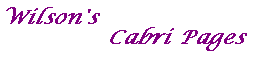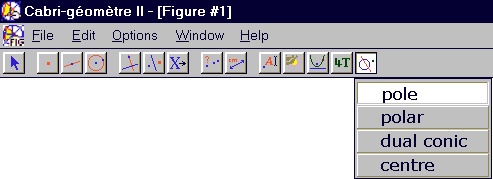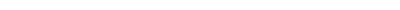pole of a line For a given conic C, each line L is the polar of some point P. Then P is the pole of L with respect to C.polar of a point Suppose that P is a fixed point and that C is a conic. It is a well-known theorem that, if a line through P meets C at A and B, then the tangents at A and B meet on a fixed line. This is called the polar of P with respect to C. If P is on C, then this line is the tangent at P. The macro constructs the polar of a point.dual conic Duality with respect to a conic C. Suppose that F is a geometrical figure composed of points and lines. Then the dual figure F' consists of the polars of the points of F, together with the poles of the lines of F. Now suppose that D is another conic. The envelope of D is a set of lines, so has a dual D' consisting of a set of points. It is a remarkable fact that D' is also a conic. See the algebra page for a proof. by algebra, or the geometry page for a geometrical version. centre of conic In the case of a central conic (ellipse or hyperbola), the centre is the intersection of the axes. The centre of a parabola is undefined.